GFG App
Open AppBrowser
Continue

# How to Annotate Bars in Grouped Barplot in Python?

A Barplot is a graph that represents the relationship between a categoric and a numeric feature. Many rectangular bars correspond to each category of the categoric feature and the size of these bars represents the corresponding value. Using grouped bar plots,  we can study the relationship between more than two features.

In Python, we can plot a barplot either using the Matplotlib library or using the seaborn library, which is a higher-level library built on Matplotlib and it also supports pandas data structures. In this article, we have used seaborn.barplot() function to plot the grouped bar plots.

Another important aspect of data visualization using bar plots is, using annotations i.e adding text for a better understanding of the chart. This can be achieved by using the annotate() function in pyplot module of matplotlib library as explained in the below steps.

Step 1: Importing the libraries and the dataset used. Here we have used the Titanic dataset, which is inbuilt with seaborn.

## Python3

 `# importing the libraries used ` `import` `seaborn as sns ` `import` `matplotlib.pyplot as plt ` ` `  `# Importing the dataset ` `df ``=` `sns.load_dataset(``"titanic"``) ` `print``(df.head()) `

Output: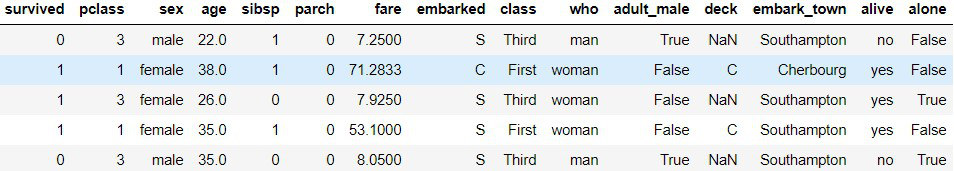Original Dataset

We will plot a grouped bar plot to analyze the average age and count of travelers on titanic, gender-wise in different ticket classes. For that, we need to transform the dataset.

Step 2: Transforming the dataset using grouping and aggregation on the original dataset.

## Python3

 `# transforming the dataset for barplot ` `data_df ``=` `df.groupby([``'sex'``, ``'class'``]).agg( ` `    ``avg_age``=``(``'age'``, ``'mean'``), count``=``(``'sex'``, ``'count'``)) ` ` `  `data_df ``=` `data_df.reset_index() ` `print``(data_df.head()) `

Output: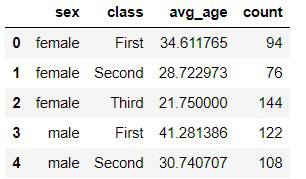transformed dataset

Explanation: Grouped bar plots require at least two categorical features and a numerical feature. Here, we have filtered out the ‘class’ feature to categorize and the ‘sex’ feature to group the bars using pandas.Dataframe.groupby() function. Then we have aggregated the mean age and count for each group using pandas.core.groupby.DataFrameGroupBy.agg(). Previous operations resulted in a multi-index dataframe, hence we reset the index to obtain the dataset shown below.

Step 3: Now, we plot a simple Barplot using the transformed dataset using seaborn.barplot() function.

## Python3

 `# code to plot a simple grouped barplot ` `plt.figure(figsize``=``(``8``, ``6``)) ` `sns.barplot(x``=``"class"``, y``=``"avg_age"``, ` `            ``hue``=``"sex"``, data``=``data_df, ` `            ``palette``=``'Greens'``) ` ` `  `plt.ylabel(``"Average Age"``, size``=``14``) ` `plt.xlabel(``"Ticket Class"``, size``=``14``) ` `plt.title(``"Simple Grouped Barplot"``, size``=``18``) `

Output :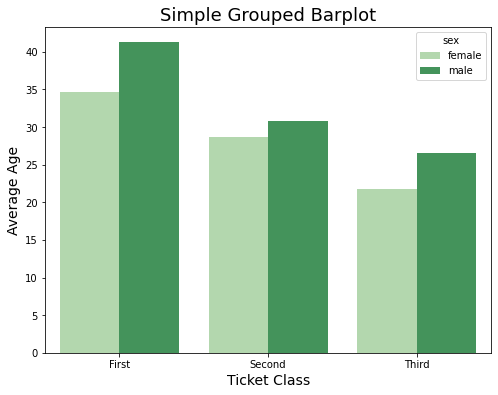Note that, We have used ‘hue’ keyword argument to group the bars based on ‘sex’ feature.

Step 4: Annotating the bars

## Python3

 `# code for annotated grouped barplot ` `plt.figure(figsize``=``(``8``, ``6``)) ` `splot ``=` `sns.barplot(x``=``"class"``, y``=``"avg_age"``, hue``=``"sex"``, ` `                    ``data``=``data_df, palette``=``'Greens'``) ` ` `  `for` `p ``in` `splot.patches: ` `    ``splot.annotate(``format``(p.get_height()), ` `                   ``(p.get_x() ``+` `p.get_width() ``/` `2.``, p.get_height()), ` `                   ``ha``=``'center'``, va``=``'center'``, ` `                   ``xytext``=``(``0``, ``9``), ` `                   ``textcoords``=``'offset points'``) ` `     `  `plt.ylabel(``"Average Age"``, size``=``14``) ` `plt.xlabel(``"Ticket Class"``, size``=``14``) ` `plt.title(``"Grouped Barplot with annotations"``, size``=``18``) `

Output: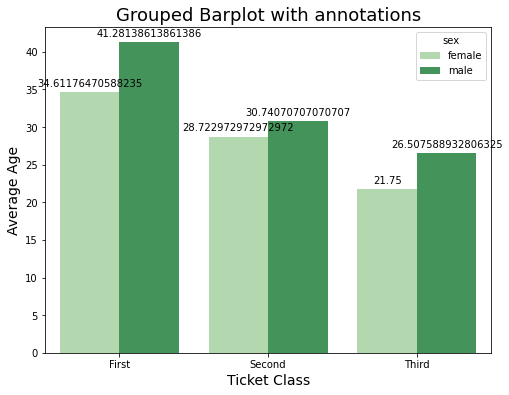Explanation: In the above code, we have used the ‘patches’ attribute of the seaborn plot object to iterate over each bar. We have calculated the height, coordinates, and put text using the annotate function for each bar.

Step 5: Since each bar represents age and putting decimal doesn’t make its value sensible. We will customize our text by rounding off to the nearest integer and then using the format() function as shown in the code below.

## Python3

 `# code for annotated barplot ` `plt.figure(figsize``=``(``8``, ``6``)) ` `splot ``=` `sns.barplot(x``=``"class"``, y``=``"avg_age"``, hue``=``"sex"``, ` `                    ``data``=``data_df, palette``=``'Greens'``) ` ` `  `plt.ylabel(``"Average Age"``, size``=``14``) ` `plt.xlabel(``"Ticket Class"``, size``=``14``) ` `plt.title(``"Grouped Barplot with annotations"``, size``=``18``) ` `for` `p ``in` `splot.patches: ` `    ``splot.annotate(``format``(``round``(p.get_height()), ``'.0f'``)``+``"Years"``, ` `                   ``(p.get_x() ``+` `p.get_width() ``/` `2.``, p.get_height()), ` `                   ``ha``=``'center'``, va``=``'center'``, ` `                   ``size``=``14``, ` `                   ``xytext``=``(``0``, ``-``12``), ` `                   ``textcoords``=``'offset points'``) `

Output: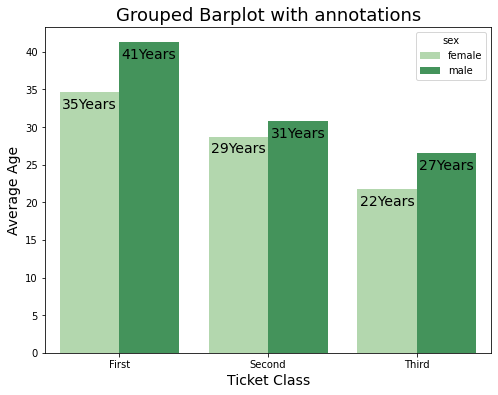Also, by changing the coordinates we have shifted our text inside the bar.

My Personal Notes arrow_drop_up